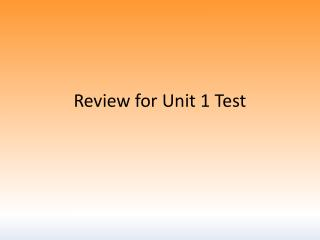DownloadDownload PresentationReview for Unit 1 Test

# Review for Unit 1 Test

Télécharger la présentation## Review for Unit 1 Test

- - - - - - - - - - - - - - - - - - - - - - - - - - - E N D - - - - - - - - - - - - - - - - - - - - - - - - - - -
##### Presentation Transcript

1. Review for Unit 1 Test

2. 1. 1 and 2 are a linear pair.1 = 5x + 252 = 4x + 20 Find m2 5x + 25 + 4x + 20 = 180 9x + 45 = 180 9x = 135 x = 15 2= 4(15) + 20 = 80

3. x + 4y – 15 + 2x + y – 10 = 6x – 5y -3x + 10y = 25 M Y is the midpoint of RL mEYL = (6x – 5y)Write and solve a system of equations to find the values of x and y. 2. YI bisects LYE R x + 4y – 15 = 2x + y – 10 -x + 3y = 5 Y L 2(x + 4y – 15) = 6x – 5y 2x + 8y – 30 = 6x – 5y -4x + 13y = 30 I E (2x + y – 10) (x + 4y – 15) 2(2x + y – 10) = 6x – 5y 4x + 2y – 20 = 6x – 5y -2x + 7y = 20 x = 25 y = 10

4. 3. Six times an angle’s supplement is 5 less than the angle. Find the measure of the angle’s supplement. 6(180 – x) = x – 5 1080 – 6x = x – 5 1085 = 7x 155 = x Supplement = 180 – 155 = 25

5. 4. 1 and 2 form a right angle.1 = 10x + 122 = 3x Find m1 10x + 12 + 3x = 90 13x + 12 = 90 13x = 78 x = 6 1= 10(6) + 12= 72

6. 5. 1 and 2 are complementary.1 is 15 more than twice 2 Find m2 2 = x; 1 = 90 – x 90 – x = 2x + 15 75 = 3x 25 = x

7. 6. 1 and 2 are vertical angles.1 = 3x2 – 2x + 62 = x2 – 11x + 2 Find the value of x. 3x2 – 2x + 6 = x2 – 11x + 2 2x2 + 9x + 4 = 0 (2x + 1)(x + 4) = 0 x = -½ x = -4 (both work!)

8. 7. The sum of an angle’s supplement and its complement is equal to 8 times the angle. Find the measure of the angle. 180 – x + 90 – x = 8x 270 – 2x = 8x 270 = 10x 27 = x

9. 8. JM intersects GW at point J GJM = 2x + 5MJW = x + 25Draw and label a diagram. G J 2x + 5 W x + 25 M

10. M Y is the midpoint of RL 9. Can you assume: YI bisects LYE R Y L I E d) R, Y, L are collinear e) Angle LYI = angle EYI a) SO + OU = SU c) Angle LYE is right b) RY = YL f) MY = YI e) Angle LYI = angle MYR d) yes e) yes, b/c you’re told BISECTOR a) yes c) no! b) yes, b/c you’re told MIDPOINT f) no! e) yes, b/c they are vertical angles

11. 10. Anangle is twice it’s complement. Find the measure of the angle’s supplement. x = 2(90 – x) x = 180 – 2x 3x = 180 x = 60 Supplement = 180 – 60 = 120

12. 11. Solve the system using either substitution or elimination. 3x + 2y = 11 -6x + y = -32 x = 5 y = -2